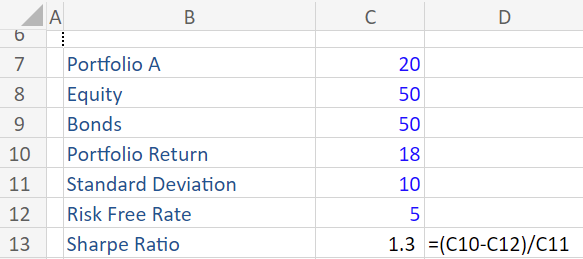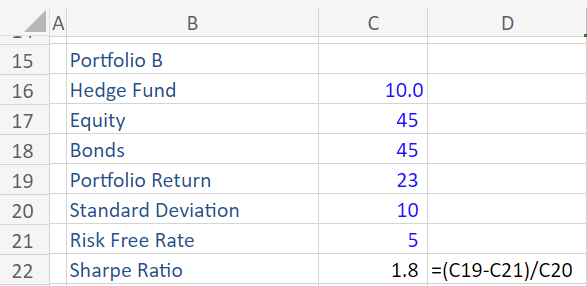## What is Sharpe Ratio?

The Sharpe ratio is a measure of the risk-adjusted return of a portfolio and is defined as a portfolio’s excess return divided by its risk (i.e. the standard deviation of portfolio return). It is used to evaluate the investment performance of a portfolio, by adjusting for its risk and relates returns to risks taken. Such a measure, being an efficiency ratio, allows portfolio managers to improve their investment decision-making by taking corrective measures. The Sharpe ratio also helps in portfolio or investment comparisons.

The Sharpe ratio is considered one of the most powerful measures for the selection of mutual funds. It helps to ascertain the degree of risk faced by two funds earning additional returns over the risk-free rate (usually the 90-day treasury bill is used as a proxy for the risk-free rate). As the Shape ratio uses standard deviation to measure a mutual fund’s risk-adjusted returns, this ratio can be used to compare the risk-adjusted returns across all fund categories.

## Key Learning Points

• To calculate the Sharpe ratio, we need to first compute the excess return and thereafter divide it by the standard deviation of portfolio return.
• A Sharpe ratio above 1 but less than 2 is considered acceptable, greater than 2 but less than three is considered very good, and above 3 is considered excellent.

## Sharpe Ratio Formula

The Sharpe ratio can be calculated using the following formula:

Sharpe Ratio = (R(P) – R(F))/Std Dev (P)

R(P) = Expected return on portfolio

R(F) = Risk-free rate of return

S(P): Standard deviation of portfolio return

The inherent risk in an investment is determined by using the standard deviation of portfolio return. The Sharpe ratio is calculated by first computing the excess return i.e. return on portfolio minus the risk-free rate of return. Thereafter, the excess return is divided by the standard deviation of the portfolio return.

The higher the Sharpe ratio of a portfolio, the better its investment performance. If the excess return (i.e. the numerator) is positive, the portfolio with the highest (lowest) Sharpe ratio has the best (worst) risk-adjusted investment performance.

Next, the Sharpe ratio is useful to explain whether the portfolio’s excess returns are a result of taking too much risk, or due to sound investment decision-making.

If there are two funds and both have similar returns, the one with the higher (lower) standard deviation of returns will have a lower (higher) Sharpe ratio. Investors prefer a Sharpe ratio that indicates a high expected return for a relatively low amount of risk.

A Sharpe ratio between 1-1.99 is considered as acceptable or good, greater than 2 is considered very good, and higher than 3 is considered excellent.

Having stated the above, the Sharpe ratio has some limitations: using standard deviation as a metric of volatility, this ratio can be manipulated by portfolio managers to enhance or boost their risk-adjusted returns and it focuses on volatility and not its direction (i.e. it cannot distinguish between the upside and the downside). Further, if used in isolation, the Sharpe ratio does not provide enough information and it uses total risk as a measure of risk when only systematic risk is priced.

## Sharpe Ratio – Example

Given below is an example of two portfolios and their respective Sharpe ratios. In this example, we assume that portfolio A consists of 50% equity and 50% bonds with a portfolio return of 18%. The standard deviation of the portfolio is 10. Further, we assume a risk-free rate of 5%. Using the aforesaid formula, we get a Sharpe Ratio of 1.3%.Now, assume that the portfolio manager wants to enhance the overall portfolio return from 18%, without adding any undue risk. So, the portfolio manager reduces the allocation to equity and bonds to 45% for each and adds an asset class that has a low or negative correlation with these assets – namely, a hedge fund.

After this asset class is added to the portfolio, we see that the portfolio return increases to 23%, while the standard deviation remains unchanged. Therefore, the Sharpe ratio increases to 1.8 i.e. when this new asset class is added to the portfolio, the overall portfolio returns increase but the risk remains the same. Consequently, the Sharpe ratio increases. Therefore, portfolio B is considered superior to portfolio A and shows the benefits of portfolio diversification.Explore the 3 other key performance measures used when assessing portfolio returns with our Portfolio Management Certification course, master the skills needed to build successful investment portfolios, and receive a recognized Wall Street Certification.